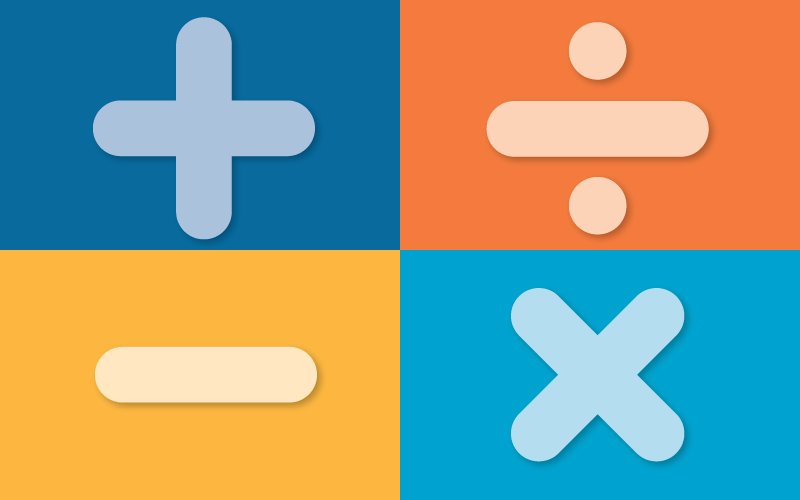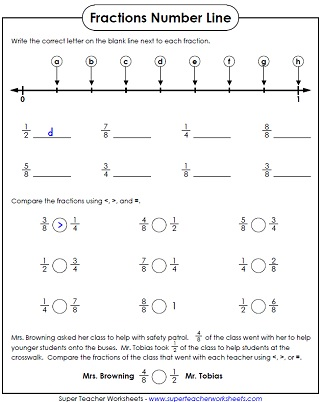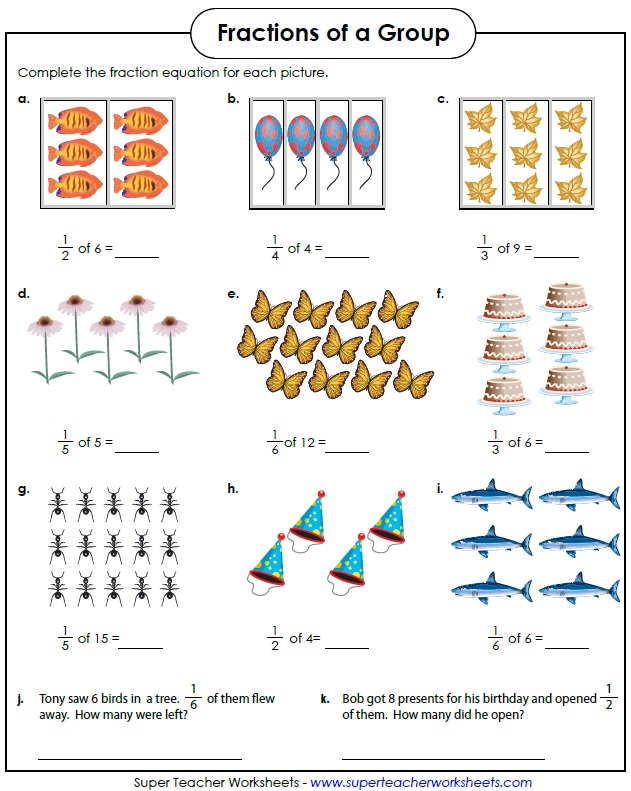Advanced fraction worksheets include equivalent fractions, ordering fractions, comparing fractions and "fractions of a number."Equivalent Fractions
Find pairs of fractions that are equivalent in this memory match game.
Fill in the missing fraction parts to make equivalent fractions.
Find equivalent fractions. This worksheet includes many graphical questions that help students to visualize the concept.
Determine the correct fraction amounts to balance the scales.
Match the fractions on the left with the equivalent fractions on the right. At the bottom, circle three fractions that are equal. This worksheet includes colorful circle illustrations.
This bookmark shows equivalent fractions, in order, from least to greatest.
Simplifying Fractions
Reduce (simplify) each fraction into its lowest terms.
Another worksheet on simplifying or reducing  fractions.
This worksheet has 5 parts. First, reduce fractions into simplest form. Then circle the fractions that are in simplest form. After that, answer two word problems. Then tell whether each equation is true or false.
This is a set of 30 task cards on reducing fractions into simplest form.
Simplify each fraction in this image. Then color the corresponding section according to the answer. Students will reveal a ladybug image as the completed math mystery picture.
Each of these word problems has a fraction answer that needs to be reduced, and written in simplest form.
Fractions of Groups
Find the fraction of each group; Includes only unit fractions; Easier version  (example: 1/5 of 15).
Find the fraction of each group; Harder version  (example: 3/7 of 28).
Use the illustrations to help find the fraction of each number; Unit fractions only.  (example: 1/3 of 9)
Color fractions of each group of marbles; Includes unit fractions only; Easier version  (example: 1/3 of 21).
Color fractions of each group of marbles; Harder version  (example: 4/7 of 21).
This file has 30 task cards with problems that require students to calculate fraction of a set. (example: 3/4 of 24).
Ordering & Counting
Write the fractions that correspond with the letters shown on the number lines.
Count by halves.  Great for helping students learn to measure inches on a ruler.
Count by fourths.  Excellent for helping students learn to measure to the nearest quarter inch on a ruler.
Place the fraction cards in order from least to greatest.
The dancing pigs have fractions on them.  Cut out the pigs and place them in order from least to greatest.
Comparing Fractions
Compare the pairs of fractions using the greater than, less than, or equal to signs.
Compare the pairs of fractions using the greater than, less than, or equal to signs.
Color the fraction circle pictures according to the instructions, then determine which is greater.
These word problems require students to compare two fractions and determine which is greater.
Here's a set of 30 task cards. Each card has two fractions on it. Students compare using the mathematical symbols <, >, and =.
Converting Fractions
Write the improper fraction for each mixed number. This worksheet also includes an improper fraction word problem.
Fraction Worksheets (Basic)

Worksheets for teaching basic fraction recognition skills and fraction concepts.

Mixed Number Worksheets

Worksheets for teaching students about mixed number concepts.

Factoring Worksheets

Worksheets for teaching basic fraction recognition skills and fraction concepts.

Add and Subtract Fractions with same and different denominators; Also add and subtract mixed numbers.

Reciprocal Fractions

We also have printable worksheets for teaching and reviewing reciprocal fractions.

Sample Pictures of
Fraction Worksheets